testlags
Perform lag exclusion (Wald) tests on a VAR.
Syntax
var_name.testlags(options)
Options

 name=arg Save the Wald test statistics in named matrix object. See below for a description of the statistics contained in the stored matrix. p Print the result of the test.
The “name=” option stores results in an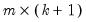matrix, where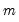is the number of lagged terms and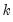is the number of endogenous variables in the VAR. In the firstcolumns, the i-th row, j-th column entry is the Wald statistic for the joint significance of all i-th lagged endogenous variables in the j-th equation. These Wald statistics have a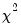distribution withdegrees of freedom under the exclusion null.
In the last column, the i-th row contains the system Wald statistic for testing the joint significance of all i-th lagged endogenous variables in the VAR system. The system Wald statistics has a chi-square distribution with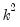degrees of freedom under the exclusion null.
Examples
var var1.ls 1 6 lgdp lm1 lcpi
freeze(tab1) var1.testlags(name=lags)
The first line declares and estimates a VAR. The second line stores the lag exclusion test results in a table named TAB1, and stores the Wald statistics in a matrix named LAGS.
Cross-references
See “Diagnostic Views” for a discussion other VAR diagnostics.